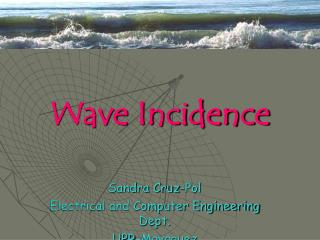DownloadDownload PresentationWave Incidence

# Wave Incidence

Télécharger la présentation## Wave Incidence

- - - - - - - - - - - - - - - - - - - - - - - - - - - E N D - - - - - - - - - - - - - - - - - - - - - - - - - - -
##### Presentation Transcript

1. Wave Incidence Sandra Cruz-Pol Electrical and Computer Engineering Dept. UPR-Mayaguez

2. Wave incidence • For many applications, like fiber optics, it’s necessary to know what happens to a wave when it meets a different medium. • How much is transmitted? • How much is reflected back?

3. We will look at… • Normal incidence Wave arrives at 0o from normal • Standing waves • Oblique incidence Wave arrives at another angle • Snell’s Law and Critical angle • Parallel or Perpendicular • Brewster angle

4. Et Ei ak Hi ak Ht Incident wave Transmitted wave Er akr Hr Reflected wave Reflection at Normal Incidence x z y z=0 Medium 2 Medium 1

5. Ei ak Hi Incident wave Now in terms of equations … • Incident wave

6. Er akr Hr Reflected wave Reflected wave • It’s traveling along –z axis

7. Et ak Ht Transmitted wave Transmitted wave

8. The total fields • At medium 1 and medium 2 • Tangential components must be continuous at the interface

9. Define • Reflection coefficient, G • Transmission coefficient, t • Note: • 1+ G= t • Both are dimensionless and may be complex • 0≤|G|≤1

10. PE 10.8 A 5GHz uniform plane wave Eis=10e-jbzax in free space is incident normally on a large plane, lossless dielectric slab (z>0) having e = 4eo and m=mo. Find: • the reflected wave Ersand • the transmitted wave Ets. Answer: -3.33 ejb1zx V/m, 6.67 e-jb2zx V/m where b2 = 2b1 = 200 p/3

11. Case 1: • Medium 1 = perfect dielectric s1=0 • Medium 2 = perfect conductor s2=∞ Hallaimpedancias int. Refleccion, Transmisión Y campos http://www.phy.ntnu.edu.tw/java/waveSuperposition/waveSuperposition.html

12. The EM field forms aStanding Wave on medium 1 |E1| 2Eio z Conducting material

13. Standing Wave Applets • http://www.phy.ntnu.edu.tw/java/waveSuperposition/waveSuperposition.html • http://www.ngsir.netfirms.com/englishhtm/StatWave.htm • http://www.physics.smu.edu/~olness/www/03fall1320/applet/pipe-waves.html

14. Case 2: • Medium 1 = perfect dielectric s1=0 • Medium 2 = perfect dielectric s2=0

15. Standing waves due to reflection |E1| Lossless Medium 1 Eio (1+|G|) z Lossless Medium 2 At every half-wavelength, everything repeats…

16. Case 3: • Medium 1 = perfect dielectric s1=0 • Medium 2 = perfect dielectric s2=0

17. Standing waves due to reflection |E1| Lossless Medium 1 Eio (1+|G|) Eio (1-|G|) z Lossless Medium 2 At every half-wavelength, all em properties repeat

18. Standing Wave Ratio, s • Measures the amount of reflections, the more reflections, the larger the standing wave that is formed. • The ratio of |E1|maxto |E1|min or Ideally s=1 (0dB) No reflections

19. PE 10.9 • The plane wave E=50 sin (wt – 5x) ay V/m in a lossless medium (m=4mo, e=eo) encounters a lossy medium (m=mo, e=4eo, s=0.1 mhos/m) normal to the x-axis at x=0. Find • G • t • s • Er • Et ←Answers: =0.8186 exp(j171o) =0.23 exp(j33.56o) =10.03 = 40.93 sin (wt +5x + 171o) y = 11.5 e -6.02x sin (wt - 7.83x + 33.6o) yV/m

20. Ex. Antenna Radome A 10GHz aircraft radar uses a narrow-beam scanning antenna mounted on a gimbal behind a dielectric radome. • Even though the radome shape is far from planar, it is approximately planar over the narrow extent of the radar beam. • If the radome material is a lossless dielectric with mr=1 and er=9, choose its thickness dsuch that the radome appears transparent to the radar beam. • Mechanical integrity requires d to be greater that 2.3 cm. Antenna with radome Antenna with no radome

21. Et Ei ak Hi ak Ht Incident wave Transmitted wave z Er y z=0 akr Hr Reflected wave Medium 2 Medium 1 Power Flow in Medium 1 • The net average power density flowing in medium 1

22. Et Ei ak Hi ak Ht Incident wave Transmitted wave z Er y z=0 akr Hr Reflected wave Medium 2 Medium 1 Power Flow in Transmitted wave • The net average power density flowing in medium 2

23. Power in Lossy Media where

24. We will look at… • Normal incidence Wave arrives at 90o from the surface • Standing waves • Oblique incidence (lossless) Wave arrives at an angle • Snell’s Law and Critical angle • Parallel or Perpendicular • Brewster angle